/

### Blending Independent Components and Principal Components Analysis 4.1 Similarities between PCA and ICA

Next page

4.1          Similarities between PCA and ICA

Despite the different scale properties of PCA and ICA there are actually many similarities. In particular, we note that the value of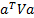for any arbitrary mixture of output signals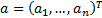of unit length, i.e. where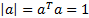is somewhere between the largest eigenvector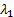and the smallest one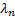(if the eigenvalues are ordered appropriately) and takes its largest value when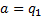. Moreover, if we remove the signal corresponding to the largest eigenvalue using Gram-Schmidt orthogonalisation then the remaining vector space is spanned by the remaining eigenvectors (all of which are orthogonal to the eigenvector being removed).

Thus PCA can be re-expressed as an example of a projection pursuit methodology but using as the importance criterion the contribution of the input signal to aggregate output signal ensemble variance rather than the magnitude of the input signal kurtosis. This explains the close analogy between methods for deciding when to stop a projection pursuit algorithm and when to truncate a PCA, i.e. random matrix theory. The two involve the same underlying mathematics, but applied to different importance criteria.

This suggests that we can blend PCA and ICA together to better capture the strengths of each, by adopting a projection pursuit type methodology applied to an importance criterion that blends together variance as well as some suitable measure(s) of independence, non-Normality and/or lack of complexity.